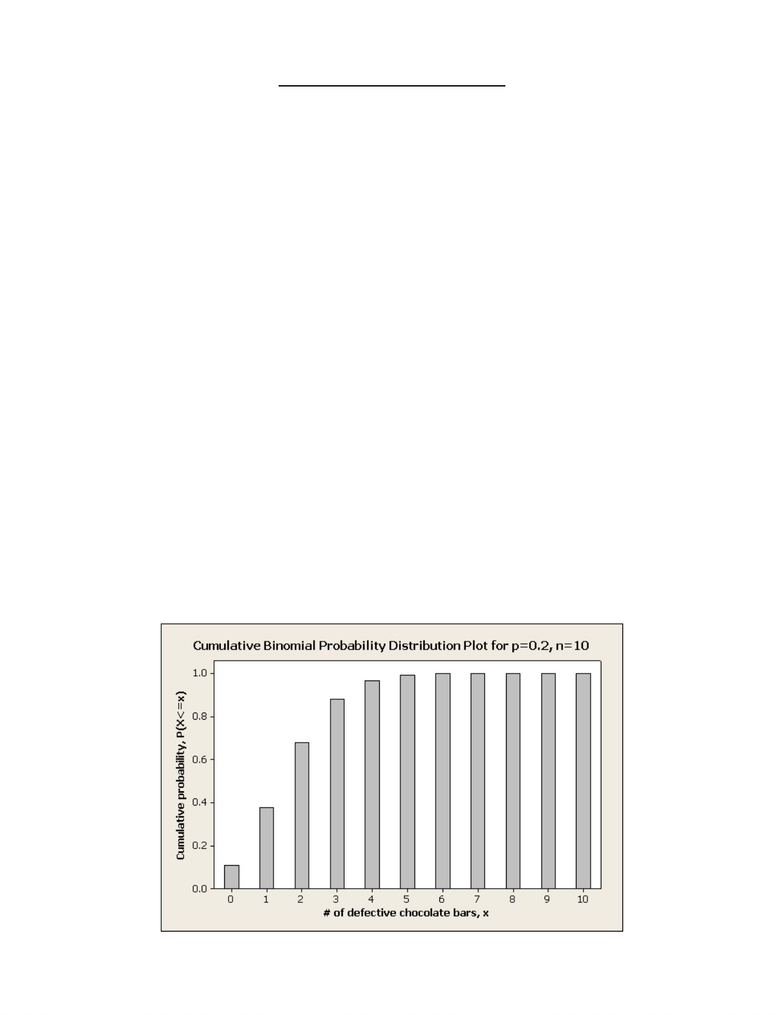# MIE231 - Minitab Tutorial 2009 V2.pdf

106 views3 pagesPage 1 of 3
MIE231 – Minitab Tutorial – 2009
Part A – Chocolate bars are packaged into boxes of 10 bars in each. The government regulation
requires that no more than X bars in a randomly packed package, where 0X10, be below a
certain mass. For the specific Binomial process at hand, the probability of a single bar being
below a certain mass (i.e., defective), p, has been estimated to be 0.2. You are asked to plot the
cumulative probability distribution for the number of defective chocolate bars in a random box.
1. Log-on to an ECF computer. Go to Start Programs MIE Software Minitab Solutions
Minitab 15 Statistical Software English.
2. You will see a Session window and a Data window (i.e., Worksheet 1). The Session window
displays the text output generated by your analyses and other work, while the Data window
displays a worksheet for data entry.
3. First, generate a list of the range of X-values over which to create the plot. On Worksheet 1,
label column C1 as “X” (type on the first row above the numbered rows). Then, go to Calc
Make Patterned Data
Simple Set of Numbers. In the resulting dialogue box, enter “X” for
the Store patterned data in input field (or double-click on C1 X in the data list on the left),
enter “0” for the From first value input field, enter “10” for the To last value input field, and
enter “1” for the In steps of input field.
4. Next, calculate the cumulative probability distribution values for each of the X values
generated above. Label column C2 as “P(X<=x)” and, then, go to Calc
Probability
Distributions
Binomial. In the resulting dialogue box, select the Cumulative probability
option and enter in the values for the number of trials and probability of success per trial in
the appropriate input fields. Also, enter “X” and “C2” for the Input column and the Optional
storage input fields, respectively.
5. To plot these cumulative distribution values, go to Graph
Bar Chart. In the Bars
represent pull-down menu select Values from a table. Select the Simple graph type and click
OK. In the resulting dialog box, enter “C2” and “X” in the Graph variables and Categorical
variable input fields, respectively. Click OK. A cumulative probability bar graph, similar to
the sample shown below, will appear.
Unlock document

This preview shows page 1 of the document.
Unlock all 3 pages and 3 million more documents.